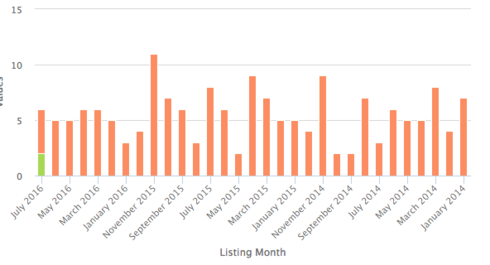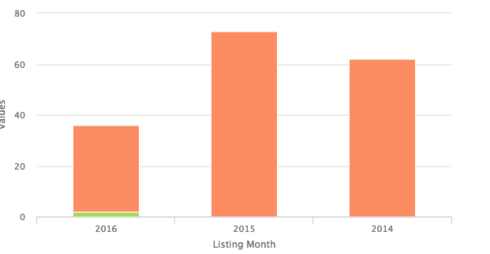# Graphs not grouping properly in Firefox or Edge

edited January 12, 2018
I have a visulization that uses two groupings on the same date field, one by calendar month and one by calendar year. It works great in Chrome, but not in Edge or Firefox

Chrome:Firefox/Edge:• Hey Jack,

Could you re-post a snapshot of what renders in Firefox/Edge? Also just curious--is there a reason why Edge or Firefox is used over Chrome?

Thanks,
Karl
• edited January 12, 2018
Hi Karl, not sure what you need, the bottom graph in the post above is what renders in Firefox and Edge. Here it is again:we are dealing with hospitals, and if they don't currently support Chrome, it will require a major security review to get it approved for use. We'd not rather not require that as a condition for our app to function.
• edited January 12, 2018
In case it helps, here's the XML for the visualization:
`<skuidvis__chart model="ListingAgg" maintitle="Patients Listed by Month" type="column" uniqueid="sk-1jCHvP-605" rendersnippet="ChangeChartLegend1" stacking="true">                                                                     <dataaxes>                                                                         <axis id="axis1"/>                                                                     </dataaxes>                                                                     <categoryaxes>                                                                         <axis id="categories" categorytype="template" field="cmListingDatec" template="{{{MonthName}}} {{{YearName}}}" title="Listing Month"/>                                                                     </categoryaxes>                                                                     <serieslist>                                                                         <series valuefield="countId" splittype="none" splittemplate="Need Pre-Transplant Audit ({{{countId}}})" splitfield="countId" aggfunction="sum"/>                                                                     </serieslist>                                                                     <colors>                                                                         <value>#fc8d62</value>                                                                         <value>#A6D854</value>                                                                     </colors>                                                                     <legend layout="horizontal" halign="center" valign="bottom"/>                                                                     <renderconditions logictype="and">                                                                         <rendercondition type="fieldvalue" enclosevalueinquotes="true" fieldmodel="ListingAgg" sourcetype="modelproperty" nosourcerowbehavior="deactivate" sourceproperty="hasRows"/>                                                                         <rendercondition fieldmodel="ListingAggComplete" sourcetype="modelproperty" nosourcerowbehavior="deactivate" sourceproperty="hasNoRows"/>                                                                     </renderconditions>                                                                 </skuidvis__chart>`
And the XML for the two models it is referencing:
`<model id="ListingAgg" limit="" query="true" createrowifnonefound="false" adapter="salesforce" type="aggregate" sobject="Listing__c" orderby="Calendar_Year(Kidney_Data__r.Listing_Date__c) DESC, Calendar_Month(Kidney_Data__r.Listing_Date__c) DESC">             <fields>                 <field id="Id" name="countId" function="COUNT"/>                 <field id="YearName" uionly="true" displaytype="FORMULA" label="YearName" readonly="true" returntype="TEXT">                     <formula>YEAR({{cyKidneyDatarListingDatec}})+1</formula>                 </field>                 <field id="MonthName" uionly="true" displaytype="FORMULA" readonly="true" returntype="TEXT" label="MonthName">                     <formula>MONTH_NAME({{cmKidneyDatarListingDatec}})</formula>                 </field>             </fields>             <conditions>                 <condition type="blank" value="null" field="Kidney_Data__c" operator="!=" enclosevalueinquotes="false"/>                 <condition type="blank" value="null" field="Kidney_Data__r.Date_of_Transplant__c" operator="=" enclosevalueinquotes="false" state="" inactive="false"/>                 <condition type="modelmerge" value="" field="Patient_Lean__r.TransplantCenter__c" operator="=" model="UserTransplantCenter" enclosevalueinquotes="true" mergefield="Id" novaluebehavior="noquery"/>                 <condition type="fieldvalue" value="" enclosevalueinquotes="false" operator="=" field="Kidney_Data__r.Listing_Date__c" state="filterableoff" inactive="true" name="Kidney_Data__r.Listing_Date__c"/>                 <condition type="fieldvalue" value="LAST_N_YEARS:2" enclosevalueinquotes="false" field="Kidney_Data__r.Listing_Date__c" operator="gte"/>                 <condition type="blank" value="null" field="Pre_Transplant_Audit_Complete__c" operator="=" enclosevalueinquotes="false"/>             </conditions>             <actions/>             <groupby method="simple">                 <field id="Kidney_Data__r.Listing_Date__c" name="cmKidneyDatarListingDatec" function="CALENDAR_MONTH"/>                 <field id="Kidney_Data__r.Listing_Date__c" name="cyKidneyDatarListingDatec" function="CALENDAR_YEAR"/>             </groupby>         </model>         <model id="ListingAggComplete" limit="" query="true" createrowifnonefound="false" adapter="salesforce" type="aggregate" sobject="Listing__c" orderby="Calendar_Year(Kidney_Data__r.Listing_Date__c) DESC, Calendar_Month(Kidney_Data__r.Listing_Date__c) DESC">             <fields>                 <field id="Id" name="countId" function="COUNT"/>                 <field id="YearName" uionly="true" displaytype="FORMULA" label="YearName" readonly="true" returntype="TEXT">                     <formula>YEAR({{cyKidneyDatarListingDatec}})+1</formula>                 </field>                 <field id="MonthName" uionly="true" displaytype="FORMULA" readonly="true" returntype="TEXT" label="MonthName">                     <formula>MONTH_NAME({{cmKidneyDatarListingDatec}})</formula>                 </field>             </fields>             <conditions>                 <condition type="blank" value="null" field="Kidney_Data__c" operator="!=" enclosevalueinquotes="false"/>                 <condition type="blank" value="null" field="Kidney_Data__r.Date_of_Transplant__c" operator="=" enclosevalueinquotes="false" state="" inactive="false"/>                 <condition type="modelmerge" value="" field="Patient_Lean__r.TransplantCenter__c" operator="=" model="UserTransplantCenter" enclosevalueinquotes="true" mergefield="Id" novaluebehavior="noquery"/>                 <condition type="fieldvalue" value="" enclosevalueinquotes="false" operator="=" field="Kidney_Data__r.Listing_Date__c" state="filterableoff" inactive="true" name="Kidney_Data__r.Listing_Date__c"/>                 <condition type="fieldvalue" value="LAST_N_YEARS:2" enclosevalueinquotes="false" field="Kidney_Data__r.Listing_Date__c" operator="gte"/>                 <condition type="blank" value="null" field="Pre_Transplant_Audit_Complete__c" operator="!=" enclosevalueinquotes="false"/>             </conditions>             <actions/>             <groupby method="simple">                 <field id="Kidney_Data__r.Listing_Date__c" name="cmKidneyDatarListingDatec" function="CALENDAR_MONTH"/>                 <field id="Kidney_Data__r.Listing_Date__c" name="cyKidneyDatarListingDatec" function="CALENDAR_YEAR"/>             </groupby>         </model>`
And also the XML for the custom snippet to adjust the chart legend:
`<jsitem location="inlinesnippet" name="ChangeChartLegend" cachelocation="false">var chartObj = arguments, \$ = skuid.\$; chartObj.series.name = 'Patients Listed';  chartObj.series.name = 'Pre-Transplant Audit Complete'; </jsitem>`